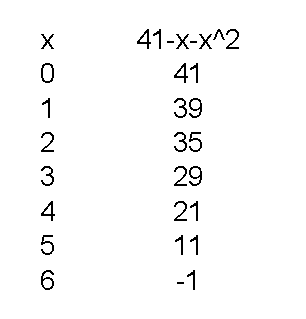# Can You Help? I Have A Formula That I Am Supposed To Use, Which Is N=-x2-x=41. I Am Suppose To Select Some Numbers For X, Substitute Them In The Formula, And See If Prime Numbers Occur. Then I Am Suppose To Try And Find A Number For X, That When I Sub It It Yields

Take a look at the picture to see your formula's value for several different x values.

For x=0, N = -(0)2 - (0) + 41 = -0-0+41 = 41.
As it happens, 41 is a prime number. You can check by dividing by odd primes less than 7, that is, 3 or 5.

For x=1, N = -(1)2 - (1) + 41 = -1-1+41 = 39.
39 is a composite number, being the product of 3 and 13.
_____
To check a number for primality, you need only divide by primes up to the square root of the number. 72=49, so all values this formula produces will require testing by only 3 and 5. Any even value other than 2 is not prime. (11, 29, 41 are prime; 21=3*7, 35=5*7, 39=3*13)thanked the writer.
Toni Flaugh commented
Thank you so very much, I did not realize this math class was going to be so hard for me, but back in my day Algebra was not a requirement to graduate(so you can guess my age is up there), but now I get it and thank you for all of your help,Toni